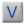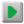# Word details

 Word: multiply (by)multiply (by)Notes: Language: English Element: verb Thesaurus: quantity: calculation Usage: occasionally Rank: 5036

# Formsmultiply (by)multiply (by) infmultiplied (by)multiplied (by) pmultiplied (by)multiplied (by) pass

# Meanings

 MS darab fiDarab fy verb ضـَر َب في

# Similar words

 EN multiply (by)multiply (by) verb